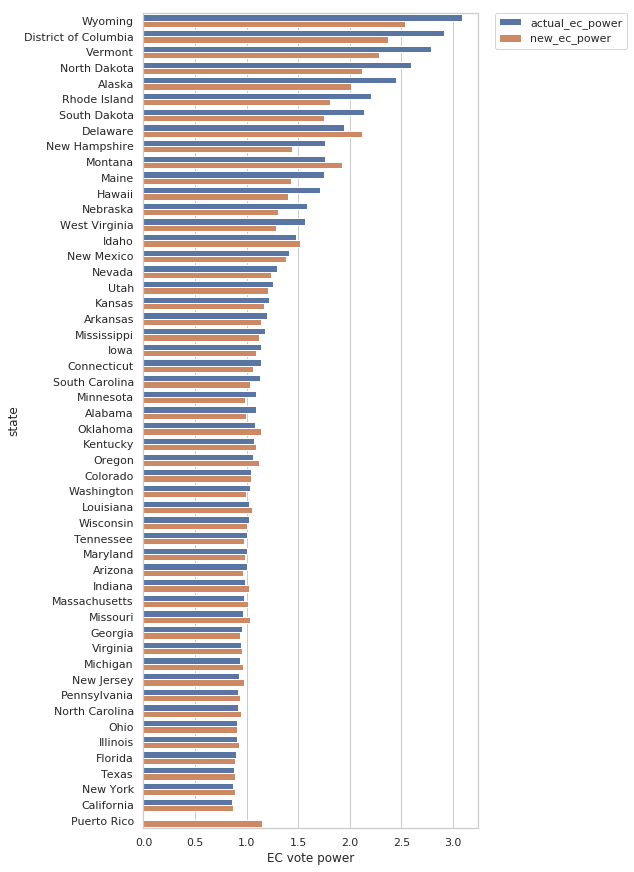# If the US House had no size limit

I used this project to test out Azure Notebooks. See the data and notebook at the project page.

It is no secret that the allocation process for US legislators between each state is quite unbalanced, assuming one is trying to give every person in the US equal representation in the legislature. Without evaluating the reasons for the current system exists, I want to investigate what the allocation would look like if we made a few changes.

First, we add in Washington, D.C. and Puerto Rico, because why not? From a population and representation standpoint, it makes sense for both of them to be states. Then we ask a few questions:

1. What happens if we relax the upper limit on the House of Representatives?
2. What if we allow the value of single representative’s vote to vary?
In :
```# TODO: scale the width of the bars in the plots by the population of the state?
```
In :
```import pandas as pd
import numpy as np
import matplotlib.pyplot as plt
import seaborn as sns

sns.set(style="whitegrid")
```

For state population data, I use the most recent national population estimates from the census, the 2018 estimates. And I get the actual numbers of representatives by state by searching and copying a table I found online; I think it was from Wikipedia.

In :
```def state_barplot_compare(data, col1, col2, xlabel):
'''Make a barplot comparing two columns in a data frame'''

f, ax = plt.subplots(figsize=(6, 15))

df_plt = data[['state', col1, col2]].copy()

df_plt.set_index('state', inplace = True)
df_plt = df_plt.stack().reset_index().rename(columns = {"level_1": "case", 0: xlabel})

sns.barplot(data = df_plt, x = xlabel, hue = "case", y = "state", orient = "h",
order = df.sort_values(col1, ascending=False)["state"])

```
In :
```# load our data
```
In :
```## combine the two datasets into a single dataframe
df = (pd.merge(df_pop.loc[df_pop["STATE"] > 0, ["NAME", "CENSUS2010POP"]], df_actual, how = "left", left_on = "NAME", right_on = "State")
.drop(columns = ["State", "Change from 2000", "Population"])
.rename(columns = {"Number of House Seats from 2010": "actual_seats",
"CENSUS2010POP": "pop",
"NAME": "state"}))
df.fillna(value = 0, inplace = True)
```

To actually figure out how many seats each state should get, I define a target constituency size. Then dividing each state’s population by that size and rounding to the nearest whole number (with a minimum of 1) gives us an approximation.

Here, I set the target constituency size to the population of the smallest state, Wyoming.

In :
```target_size = df["pop"].min()

df["prop_of_pop"] = df["pop"]/df["pop"].sum()
df["pop_rel_to_min"] = df["pop"]/target_size

df["new_seats"] =  np.round(df["pop_rel_to_min"].apply(lambda x: np.max([x, 1])))
df["new_rel_to_actual"] = (df["new_seats"]/df["new_seats"].sum())/(df["actual_seats"]/df["actual_seats"].sum())-1
df["actual_rel_to_new"] = (df["actual_seats"]/df["actual_seats"].sum())/(df["new_seats"]/df["new_seats"].sum())-1

df['actual_seats_per_pop'] = df['actual_seats']/df['pop']
df['new_seats_per_pop'] = df['new_seats']/df['pop']

## if we wanted an adjustment for the error introduced by the fact that seats are a discrete quantity

## add in senate and DC weirdness to get current electoral college
df.loc[(df['state'] == "Puerto Rico"), 'actual_ec_adj'] = np.inf
df.loc[(df['state'] == "District of Columbia"), 'actual_ec_adj'] = df['prop_of_pop']*((df['actual_seats'].sum()+100)/3)

## (state_seats/total_seats)* = vote_power*prop_of_pop
## vote_power = (state_seats/total_seats)/prop_of_pop

df['power_change'] = df['new_power'] - df['actual_power']

```

First, I just look at how the proportion of seats a state has changed. Most states decreased their proportion of representation in the House. A few increased significantly, however, especially Puerto Rico and District of Columbia.

Note that, in the change in relative representation, the values from D.C. and Puerto Rico are not 0, they’re essentially infinite, as neither has any current representation.

In :
```state_barplot_compare(df, "actual_seats", "new_seats", "# of seats")

f, ax = plt.subplots(figsize=(6, 15))
sns.set_color_codes("pastel")
sns.barplot(x="new_rel_to_actual", y="state", data=df,
label="change", color="b",
orient="h", order = df.sort_values("new_rel_to_actual")["state"])
plt.title("change in relative representation in the House")
```
Out:
`Text(0.5, 1.0, 'change in relative representation in the House')`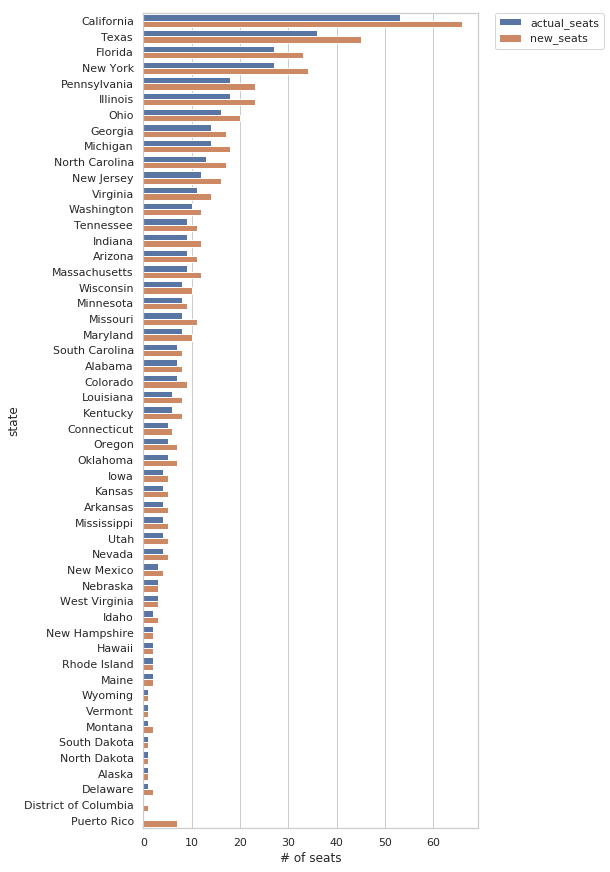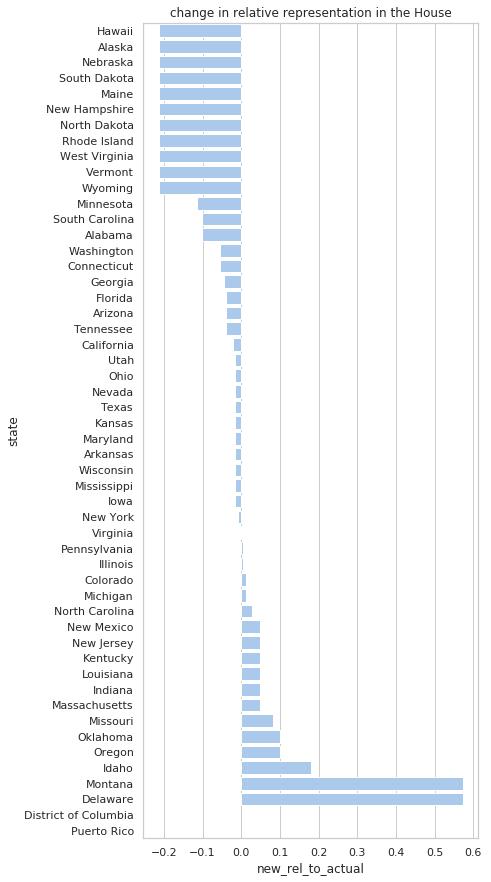The number of seats per capita in a state basically shows how much representation an individual nominally has in the House.

In :
```state_barplot_compare(df, "actual_seats_per_pop", "new_seats_per_pop", "seats per pop")
```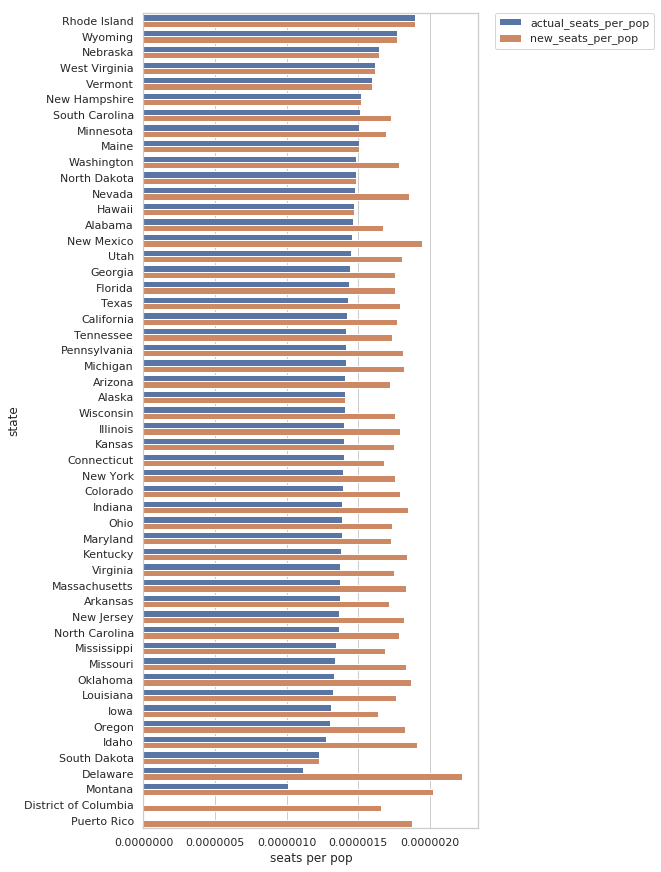I calculate an multplier for how much a representatives vote should be adjusted in order to give every person the same value of representation in the House. A value greater than 1 indicates that the state’s residents are underrepresented. This is not quite an apples-to-apples comparison, as Puerto Rico and D.C. can’t be adjusted.

In :
```state_barplot_compare(df, "actual_adj", "new_adj", "adjustment")
```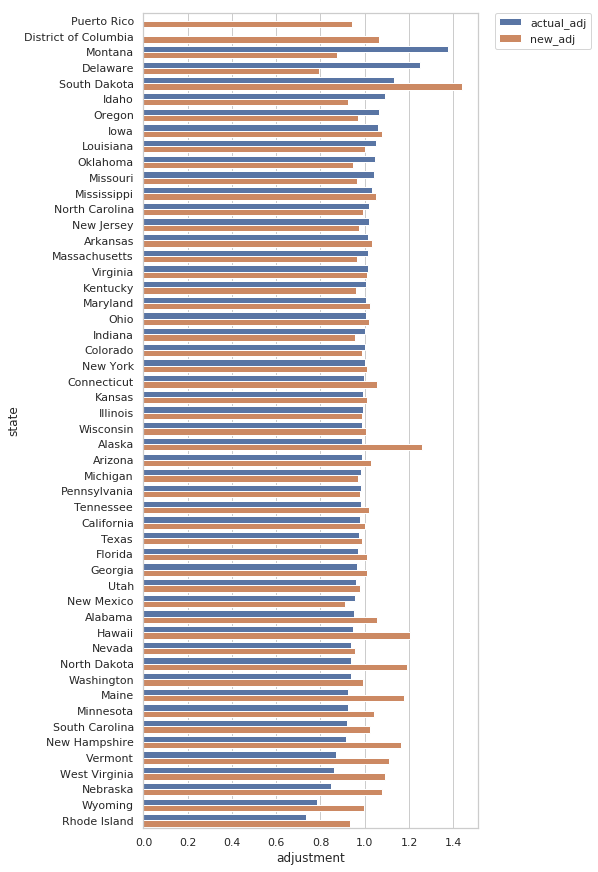The inverse of the vote adjustment can be seen as a sort of vote power, or representation power. A value greater than 1 indicates that a given state’s residents enjoy representation in the House that is greater than the average citizen’s.

Mostly, we see all the large states at about even and the smaller states being either over- or under-represented, based on how the discretization of seats happened to fall (rounding up or rounding down).

In :
```state_barplot_compare(df, "actual_power", "new_power", "vote power")

f, ax = plt.subplots(figsize=(6, 15))
sns.set_color_codes("pastel")
sns.barplot(x=df["new_power"]-df["actual_power"], y=df["state"],
label="change", color="b",
orient="h", order = df.sort_values("power_change", ascending=False)["state"])
plt.title('change in power')
```
Out:
`Text(0.5, 1.0, 'change in power')`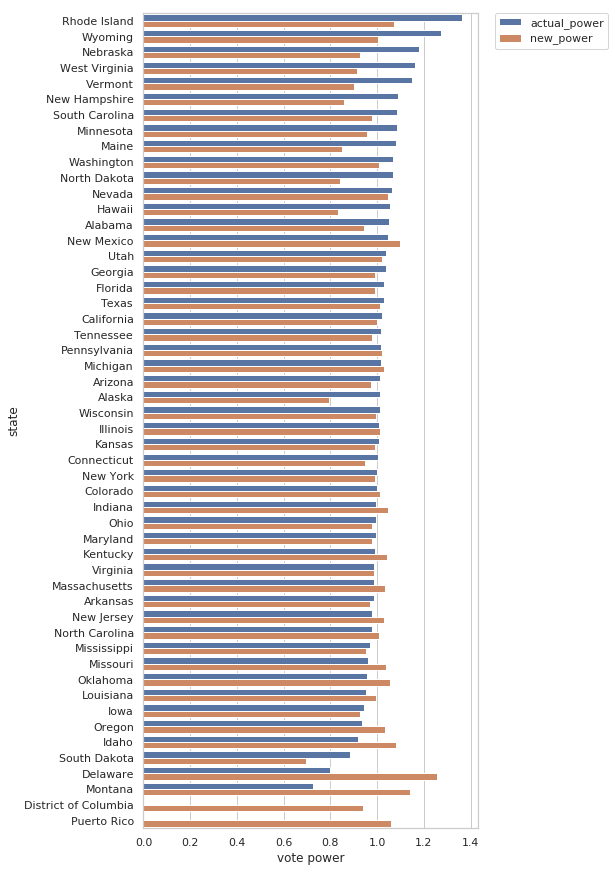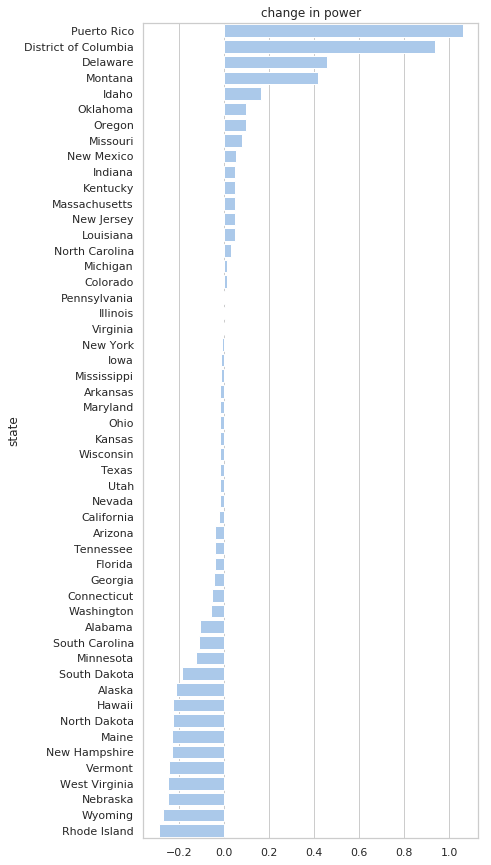Then, just for fun, we can look at how much representation an individual has in the presidential election, via the electoral college (which includes the senate and, in the current system, 3 votes for D.C.).

Basically, the overall trend of small states having extra influence due to the Senate doesn’t change, but it gets damped down a bit with the addition of a bunch of representatives. It would be better if we just got rid of that strange custom entirely…

In :
```state_barplot_compare(df, "actual_ec_power", "new_ec_power", "EC vote power")
```